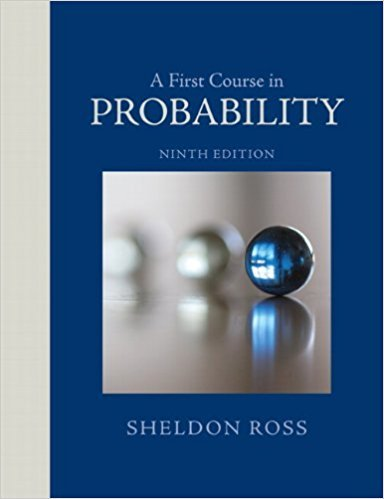×
Get Full Access to A First Course In Probability - 9 Edition - Chapter 4 - Problem 19ste
Get Full Access to A First Course In Probability - 9 Edition - Chapter 4 - Problem 19ste

×

# When three friends go for coffee, they decide who will payISBN: 9780321794772 63

## Solution for problem 19STE Chapter 4

A First Course in Probability | 9th Edition

• Textbook Solutions
• 2901 Step-by-step solutions solved by professors and subject experts
• Get 24/7 help from StudySoup virtual teaching assistantsA First Course in Probability | 9th Edition

4 5 1 389 Reviews
25
2
Problem 19STE

Problem 19STE

When three friends go for coffee, they decide who will pay the check by each flipping a coin and then letting the “odd person” pay. If all three flips produce the same result (so that there is no odd person), then they make a second round of flips, and they continue to do so until there is an odd person. What is the probability that

(a) exactly 3 rounds of flips are made?

(b) more than 4 rounds are needed?

Step-by-Step Solution:

Solution :

Step 1 of 2:

Given when 3 coins are flipped, there are 8 cases or  in 3 flips of coin possible outcomes are.

Then the probability when no odd person is there =So the probability when no odd person is there =Our goal is:

a). We need to find the probability of exactly 3 rounds of flips are made.

b). We need to find the probability of more than 4 rounds are needed.

a). The probability of exactly 3 rounds of flips are made is

P( exactly 3 rounds of flips) =P( exactly 3 rounds of flips) =P( exactly 3 rounds of flips) =P( exactly 3 rounds of flips) = 0.04685

Therefore, the probability of exactly 3 rounds of flips are made is 0.04685.

Step 2 of 2

##### ISBN: 9780321794772

Unlock Textbook Solution